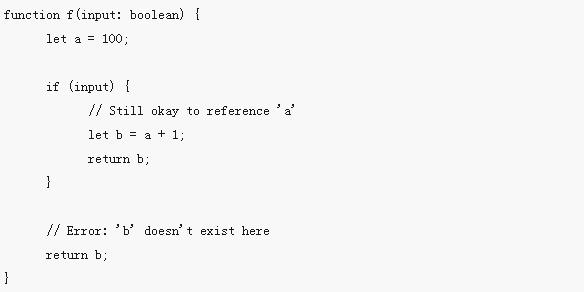# 变量声明let和const是JavaScript里相对较新的变量声明方式。 像我们之前提到过的，let在很多方面与var是相似的，但是可以帮助大家避免在JavaScript里常见一些问题。const是对let的一个增强，它能阻止对一个变量再次赋值。血雨探花 更新时间：2019-03-25 11:23:50

# 变量声明

`let``const`是JavaScript里相对较新的变量声明方式。 像我们之前提到过的，`let`在很多方面与`var`是相似的，但是可以帮助大家避免在JavaScript里常见一些问题。`const`是对`let`的一个增强，它能阻止对一个变量再次赋值。

# `var` 声明

`var a = 10;`

```function f() {
var message = "Hello, world!";

return message;
}```

```function f() {
var a = 10;
return function g() {
var b = a + 1;
return b;
}
}

var g = f();
g(); // returns 11;```

```function f() {
var a = 1;

a = 2;
var b = g();
a = 3;

return b;

function g() {
return a;
}
}

f(); // returns 2```

## 作用域规则

```function f(shouldInitialize: boolean) {
if (shouldInitialize) {
var x = 10;
}

return x;
}

f(true);  // returns '10'
f(false); // returns 'undefined'```

```function sumMatrix(matrix: number[][]) {
var sum = 0;
for (var i = 0; i < matrix.length; i++) {
var currentRow = matrix[i];
for (var i = 0; i < currentRow.length; i++) {
sum += currentRow[i];
}
}

return sum;
}```

## 捕获变量怪异之处

```for (var i = 0; i < 10; i++) {
setTimeout(function() { console.log(i); }, 100 * i);
}```

```10
10
10
10
10
10
10
10
10
10```

```0
1
2
3
4
5
6
7
8
9```

```for (var i = 0; i < 10; i++) {
// capture the current state of 'i'
// by invoking a function with its current value
(function(i) {
setTimeout(function() { console.log(i); }, 100 * i);
})(i);
}```

# `let` 声明

`let hello = "Hello!";`

## 块作用域

```function f(input: boolean) {
let a = 100;

if (input) {
// Still okay to reference 'a'
let b = a + 1;
return b;
}

// Error: 'b' doesn't exist here
return b;
}```

`catch`语句里声明的变量也具有同样的作用域规则。

```try {
throw "oh no!";
}
catch (e) {
console.log("Oh well.");
}

// Error: 'e' doesn't exist here
console.log(e);```

```a++; // illegal to use 'a' before it's declared;
let a;```

```function foo() {
// okay to capture 'a'
return a;
}

// 不能在'a'被声明前调用'foo'
// 运行时应该抛出错误
foo();

let a;```

## 重定义及屏蔽

```function f(x) {
var x;
var x;

if (true) {
var x;
}
}```

```let x = 10;
let x = 20; // 错误，不能在1个作用域里多次声明`x````

```function f(x) {
let x = 100; // error: interferes with parameter declaration
}

function g() {
let x = 100;
var x = 100; // error: can't have both declarations of 'x'
}```

```function f(condition, x) {
if (condition) {
let x = 100;
return x;
}

return x;
}

f(false, 0); // returns 0
f(true, 0);  // returns 100```

```function sumMatrix(matrix: number[][]) {
let sum = 0;
for (let i = 0; i < matrix.length; i++) {
var currentRow = matrix[i];
for (let i = 0; i < currentRow.length; i++) {
sum += currentRow[i];
}
}

return sum;
}```

## 块级作用域变量的获取

```function theCityThatAlwaysSleeps() {
let getCity;

if (true) {
let city = "Seattle";
getCity = function() {
return city;
}
}

return getCity();
}```

`let`声明出现在循环体里时拥有完全不同的行为。 不仅是在循环里引入了一个新的变量环境，而是针对每次迭代都会创建这样一个新作用域。 这就是我们在使用立即执行的函数表达式时做的事，所以在`setTimeout`例子里我们仅使用`let`声明就可以了。

```for (let i = 0; i < 10 ; i++) {
setTimeout(function() {console.log(i); }, 100 * i);
}```

```0
1
2
3
4
5
6
7
8
9```

# `const` 声明

`const` 声明是声明变量的另一种方式。

`const numLivesForCat = 9;`

```const numLivesForCat = 9;
const kitty = {
name: "Aurora",
numLives: numLivesForCat,
}

// Error
kitty = {
name: "Danielle",
numLives: numLivesForCat
};

// all "okay"
kitty.name = "Rory";
kitty.name = "Kitty";
kitty.name = "Cat";
kitty.numLives--;```

# 解构

Another TypeScript已经可以解析其它 ECMAScript 2015 特性了。 完整列表请参见 the article on the Mozilla Developer Network。 本章，我们将给出一个简短的概述。

## 解构数组

```let input = [1, 2];
let [first, second] = input;
console.log(first); // outputs 1
console.log(second); // outputs 2```

```first = input;
second = input;```

```// swap variables
[first, second] = [second, first];```

```function f([first, second]: [number, number]) {
console.log(first);
console.log(second);
}
f(input);```

```let [first, ...rest] = [1, 2, 3, 4];
console.log(first); // outputs 1
console.log(rest); // outputs [ 2, 3, 4 ]```

```let [first] = [1, 2, 3, 4];
console.log(first); // outputs 1```

`let [, second, , fourth] = [1, 2, 3, 4];`

## 对象解构

```let o = {
a: "foo",
b: 12,
c: "bar"
};
let { a, b } = o;```

`({ a, b } = { a: "baz", b: 101 });`

```let { a, ...passthrough } = o;
let total = passthrough.b + passthrough.c.length;```

### 属性重命名

`let { a: newName1, b: newName2 } = o;`

```let newName1 = o.a;
let newName2 = o.b;```

`let {a, b}: {a: string, b: number} = o;`

### 默认值

```function keepWholeObject(wholeObject: { a: string, b?: number }) {
let { a, b = 1001 } = wholeObject;
}```

## 函数声明

```type C = { a: string, b?: number }
function f({ a, b }: C): void {
// ...
}```

```function f({ a, b } = { a: "", b: 0 }): void {
// ...
}
f(); // ok, default to { a: "", b: 0 }```

```function f({ a, b = 0 } = { a: "" }): void {
// ...
}
f({ a: "yes" }); // ok, default b = 0
f(); // ok, default to {a: ""}, which then defaults b = 0
f({}); // error, 'a' is required if you supply an argument```

## 展开

```let first = [1, 2];
let second = [3, 4];
let bothPlus = [0, ...first, ...second, 5];```

```let defaults = { food: "spicy", price: "\$\$", ambiance: "noisy" };
let search = { ...defaults, food: "rich" };```

`search`的值为`{ food: "rich", price: "\$\$", ambiance: "noisy" }`。 对象的展开比数组的展开要复杂的多。 像数组展开一样，它是从左至右进行处理，但结果仍为对象。 这就意味着出现在展开对象后面的属性会覆盖前面的属性。 因此，如果我们修改上面的例子，在结尾处进行展开的话：

```let defaults = { food: "spicy", price: "\$\$", ambiance: "noisy" };
let search = { food: "rich", ...defaults };```

```class C {
p = 12;
m() {
}
}
let c = new C();
let clone = { ...c };
clone.p; // ok
clone.m(); // error!```

php中文网
phpstudy

QQ交流群